# 基于拓扑结构的互穿校正方法

Blacklock等人（2012）描述了使用新型的马尔可夫链算法生成1D丝束基因座。在这项研究中，我们采用相同的算法来生成3D丝束表示，将增广马尔可夫链算法与拓扑规则相结合以消除通过蒙特卡罗方法生成随机3D物体时不可避免出现的互穿或其他排序错误，将其转换为物理上有效的虚拟样本。# 1. 离散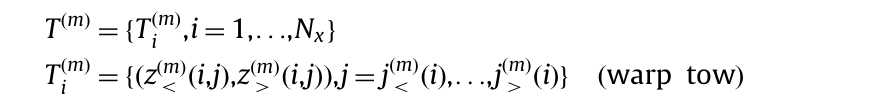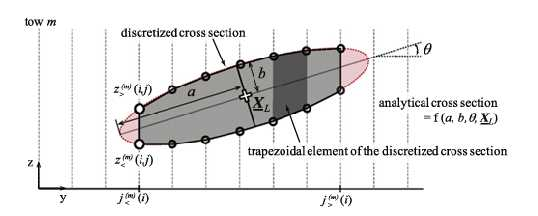# 2. Z/\rho-排序

## 2.1 Z-排序

z方向（通过厚度）上的顺序被指定为逻辑表达式的数组O(z)，任何丝束对m和n的排序可以取以下三个值：

O_{mn}^{(z)}=\begin{cases}Z_m\geq Z_n&\text{if }\Omega_m\neq\Omega_n\text{ and }m\text{ above }n\\Z_m\leq Z_n&\text{if }\Omega_m\neq\Omega_n\text{ and }m\text{ below }n\\{indeterminate}&\text{if }\Omega_m=\Omega_n\end{cases}\quad(m=1,\ldots,N_t;n=1,\ldots,N_t)## 2.2 平面内排列（\rho排列）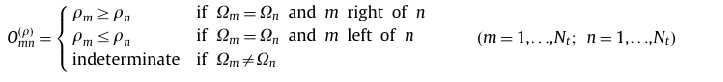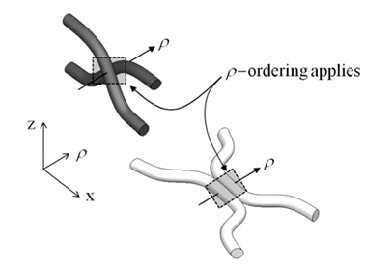## 2.3 排序符号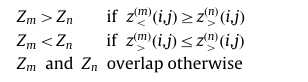# 3. 消除渗透-强制执行拓扑顺序

## 3.1 边缘后退算法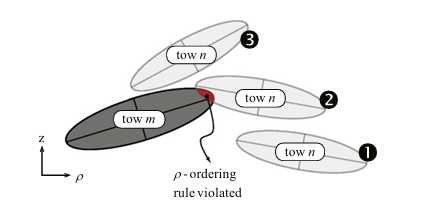(i) 第i行上任何j的间隔[z_{}^{(m)}(i,j)]\mathrm{and}[z_{}^{(n)}(i,j)]都重叠。

(ii) 丝束 m和n在行i上共享公共网格点，并且在行i上任何点j处的间隔[z_{}^{(m)}(i,j)]\mathrm{and}[z_{}^{(n)}(i,j)]的排序不同于在行i+1或行i-1上任何点j0处间隔[z_{}^{(m)}(i\pm1,j^{\prime})]\mathrm{and}[z_{}^{(n)}(i\pm1,j^{\prime})]的排序，其中排序的差异是通过考虑上述排序（2.3）中定义的三种状态来确定的。

1. 取同一属的任何一对丝束m和n。考虑两个经向丝束的例子，它们名义上与x轴对齐。首先，沿着x方向进行扫描，搜索两个丝束的截面的相互穿透。当网格点(i,j)处丝束在z方向上占据的间隔[z_{}^{(m)}(i,j)]\mathrm{and}[z_{}^{(n)}(i,j)]。网格第i行上的典型互穿情况如下图a所示。

2. 边缘后退算法消除网格行i的j重叠，直到极限点相等，并且剩余丝束截面服从排序\rho_{m}\leq\rho_{n}。网格点i的j值被选择为其旧值的平均值（\Bigg(j_{>}^{(m)}(i)-j_{<}^{(n)}(i)\Bigg)/2)，如果必要，则转移到下一个更高的整数值。在一个方向上一致地进行偏移可以最小化得到的纱线几何形状的粗糙度。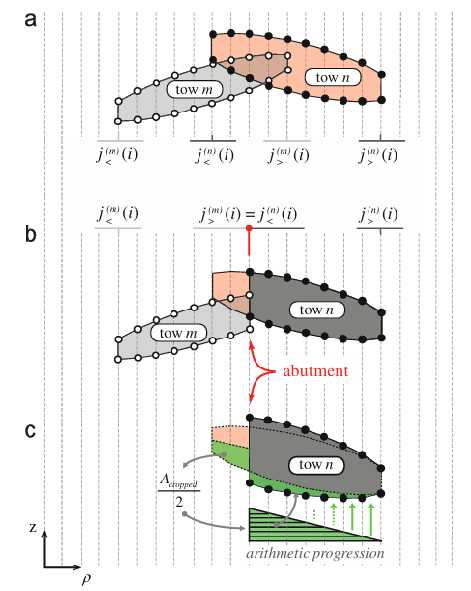(a) 边缘后退之前。 (b) 边缘后退之后，剩余的共同网格点被标记为两个纤维束之间的对接点。(c) 经过线性变化扩张后重新塑造的纤维束以保持截面积不变。

## 3.2 Z排序算法

O^{(z)}中的排序规则适用于不同属丝束的纤维束对，而相同属丝束的纤维束对的排序是不确定的。如果S_{\mathrm{i}j} 中的所有纤维束属于不同的属，它们之间的逐对排序为该网格点上的唯一整体排序。（在 S_{\mathrm{i}j}中出现冲突的逐对排序的情况几乎从不发生。它们需要同一纤维束的多个不同部分共同占据一个网格点，这只有在纤维束的横截面上具有凹入特征的情况下才可能发生。然后这种凹入特征在示例材料中从未见过。)

(1) 在S_{\mathrm{i}j}上执行成对搜索以识别排序错误中涉及的所有丝束。然后将这些丝束收集到S_{\mathrm{i}j}的子集中，表示为S_{ij}^{(p)},p=1,\ldots,并且定义为使得任何子集内的丝束彼此呈现排序误差，但不与不在该子集中的任何丝束呈现排序误差。这种分离将校正过程分解为处理例如靠近区段顶部的丝束和靠近底部的丝束的校正。

(2) 然后在每个子集内执行排序校正，调用O^{(z)}

• 如果S_{ij}^{(p)}不包含两个相同属的丝束，则S_{ij}^{(p)}排序的结果是唯一的。
• 如果S_{ij}^{(p)}包含两个相同属的丝束，则在S_{ij}^{(p)}中必然也至少存在一个不同属的丝束（否则就不会有排序错误）。如果另一个这样的丝束被排序在相同属对中的一个之上和另一个之下，则通过调用O^{(z)}进行排序的结果是唯一的。但是，如果不同属的所有丝束的排序高于或低于同一属的两个丝束，则同一属对的排序仍然是不确定的。在这种情况下，它们相对于彼此的顺序保持不变。

(3) 步骤（2）的结果是S_{ij}^{(p)}中丝束的修订排序，表示为索引整数的有序列表。为了完成排序校正，对于正确排序并相互接触的所有m\in S_{ij}^{(p)}，必须在上为丝束分配新的区间（共享共同的z区间边界)。

(4) 在分配了新的间隔之后，在两个不同的子集S_{ij}^{(p)}之间可能出现新的重叠。通过使两个重叠的集合成为单个集合并重新应用步骤（1）-（3)，利用快速收敛来消除这些。

# 4. 几何形状的调整

## 4.1 边缘撤退后丝束横截面的调整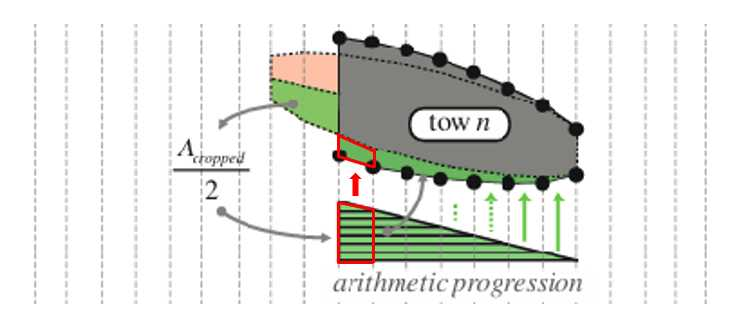## 4.2 Z排序后丝束横截面的调整

(1) 间隔是连续的，即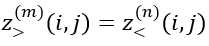，其中m和n是校正顺序中的连续间隔。

(2) 每个间隔的长度，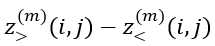，不变。

(3) 间隔的质心不变，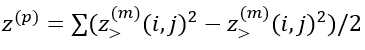，其中和是在S_{ij}^{(p)}上得出的。